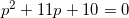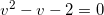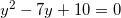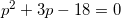### Day 25 - Factoring Trinomials - 02.12.15

• Unit 3 Test next Friday, February 20th!

Bell Ringer

1. Solve1. -10

2. -1

3. 10

4. 1

5. none of the above

2. Solve1. 1

2. -1

3. 2

4. -2

5. none of the above

3. Solve1. 2

2. -2

3. 5

4. -5

5. none of the above

4. Solve1. 3

2. -3

3. 6

4. -6

5. none of the above

Review
• Prerequisites
• Prime Numbers
• Composite Numbers
• Factor
• Distributive Property
• Factoring (video 1) (video 2)
• Classifying Numbers as Prime or Composite
• Prime Factorization of a Positive Integer
• Prime Factorization of a Negative Integer
• Prime Factorization of a Monomial
• Finding the GCF of an Integer
• Finding the GCF of a Monomial
• Apply Factoring to Solve Problems
• Factoring and Distributive Property (video)
• Zero Product Property (video)
• Factor polynomials using the Distributive Property
• Factor by grouping
• Solve quadratic equations of the following form:

Lesson

Exit Ticket
• Posted on board at the end of the block
Lesson Objective(s)
• How can trinomials of the form   be factoring?
• How can trinomial equations of the form  be solved?
Skills
• Factor trinomials of the form
• Solve equations of the form
• Factor trinomials of the form
• Solve equations of the form

#### In-Class Help Requests

Standard(s)
• CC.9-12.A.SSE.1a Interpret parts of an expression, such as terms, factors, and coefficients.

Mathematical Practice(s)
• #1 - Make sense of problems and persevere in solving them
• #2 - Reason abstractly and quantitatively
• #7 - Look for and make use of structure

Past Checkpoints

Unit Skills
1. Classifying Numbers as Prime or Composite
2. Prime Factorization of a Positive Integer
3. Prime Factorization of a Negative Integer
4. Prime Factorization of a Monomial
5. Finding the GCF of an Integer
6. Finding the GCF of a Monomial
7. Factor Applications
8. Factor polynomials using the Distributive Property
9. Factor by grouping
10. Solve quadratic equations of the following form: